# Exercise 15.1 Probability NCERT Solutions Class 9

## Chapter 15 Ex.15.1 Question 1

In a cricket match, a batswoman hits a boundary $$6$$ times out of $$30$$ balls she plays. Find the probability that she did not hit a boundary.

### Solution

What is known?

Total number of balls played and number of times the batswoman hits boundary.

What is unknown?

Probability of the batswoman not hitting a boundary.

Reasoning:

If the batswoman hits a boundary $$6$$ times out of $$30$$ balls then she will not hit a boundary in the remaining $$24$$ balls.

The empirical probability $$P(E)$$ of an event $$E$$ happening, is given by:

\begin{align}{P}({E})=\frac{ \begin{bmatrix} \text { Number of trials in which }\\ \text{the event happened } \end{bmatrix} }{ \begin{bmatrix} \text { The total number} \\ \text{of trials } \end{bmatrix} }\end{align}

Steps:

Number of balls played $$= 30$$

Number of balls the batswoman hits boundary $$= 6$$

Number of balls the batswoman does not hit a boundary $$= 30 - 6 = 24$$

$$P$$(bats - woman does not hit the boundary)
\begin{align} & = \frac{ \begin{bmatrix}\text{Number of times the bats-woman}\\ \text{ does not hit the boundary} \end{bmatrix}} {\text{Number of balls played}} \\ & = 24/30 \\ &= 4/5 \end{align}

## Chapter 15 Ex.15.1 Question 2

$$1500$$ families with $$2$$ children were selected randomly, and the following data were recorded:

 Number of girls in a family $$2$$ $$1$$ $$0$$ Number of families $$475$$ $$814$$ $$211$$

Compute the probability of a family, chosen at random, having

(i) $$2$$ girls

(ii) $$1$$ girl

(iii) No girl

Also check whether the sum of these probabilities is $$1.$$

### Solution

What is known?

Number of families having $$2$$ girl child,$$1$$ girl child, no girl child and total number of families.

What is the unknown?

Probability of selecting family with $$2$$ or $$1$$ or $$0$$ girls.

Reasoning:

The empirical probability $$P(E)$$ of an event $$E$$ happening, is given by:

\begin{align}{P}({E})=\frac{ \begin{bmatrix} \text { Number of trials in which }\\ \text{the event happened } \end{bmatrix} }{ \begin{bmatrix} \text { The total number} \\ \text{of trials } \end{bmatrix} }\end{align}

Use probability to derive the solution where

Probability of an selecting family

\begin{align} & { = \frac{{\text{Family having 2 or 1 }} {\text{or 0 girl child}} } {{{\text{Total number of families}}}}} \end{align}

Steps:

No of families having $$2$$ girl child $$= 475$$

No of families having $$1$$ girl child $$= 814$$

No of families having $$0$$ girl child $$= 211$$

Therefore, Total number of families $$= 1500$$

Probability $$P1$$ [family having $$2$$ girl child]

\begin{align} & { = \frac{{\text{Family having }} {\text{ 2 girl child}} } {{{\text{Total number of families}}}}} \end{align}

\begin{align}\ \therefore Pl=\frac{475}{1500}=\frac{19}{60}\end{align}

Probability $$P2$$ [family having $$1$$ girl child]

\begin{align} & { = \frac{{\text{Family having }}{\text{ 1 girl child}} } {{{\text{Total number of families}}}}} \end{align}

\begin{align} \therefore P2=\frac{814}{1500}=\frac{407}{750}\end{align}

Probability $$P3$$ [family having $$0$$ girl child]

\begin{align} & { = \frac{{\text{Family having }} {\text{ 0 girl child}} } {{{\text{Total number of families}}}}} \end{align}

\begin{align} \therefore P3=\frac{211}{1500}\end{align}

Sum of all the three probabilities

\begin{align} &={P1}+{P2} +{P3} \\ &=\frac{475}{1500}+\frac{814}{1500}+\frac{211}{1500} \\ &=\frac{1500}{1500} \\ &=1 \end{align}

## Chapter 15 Ex.15.1 Question 3

Refer to Example 5, Section 14.4, Chapter 14. Find the probability that a student of the class was born in August.

In a particular section of Class IX, $$40$$ students were asked about the months of their birth and the following graph was prepared for the data so obtained. Find the probability that a student of the class was born in August.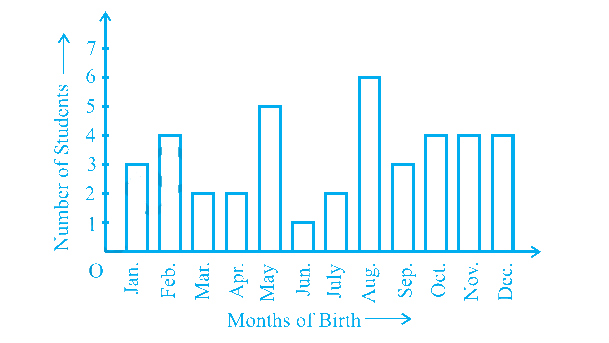### Solution

What is known?

Total number of students in class and number of students born in each month.

What is unknown?

Probability of students born in the month of August.

Reasoning:

The empirical probability $$P(E)$$ of an event $$E$$ happening, is given by:

\begin{align}{P}({E})=\frac{ \begin{bmatrix} \text { Number of trials in which }\\ \text{the event happened } \end{bmatrix} }{ \begin{bmatrix} \text { The total number} \\ \text{of trials } \end{bmatrix} }\end{align}

Use probability to derive the solution where

Probability [students born in particular month]

\begin{align} & = \frac{\begin{bmatrix}{\text{No of students born }} \\ {\text{in particular month} }\end{bmatrix}}{\begin{bmatrix} \text{Total number of} \\\text{ students in class} \end{bmatrix}} \end{align}

Steps:

Given total number of students in class $$= 40$$

No of students born in August $$= 6$$

Probability students born in August

\begin{align} & { = \frac{\begin{bmatrix} {\text{No of students born in }} \\ {\text{ the }} {\text{month of August}}\end{bmatrix} } { \begin{bmatrix} \text{Total number of}\\ \text{ students in class } \end{bmatrix}} } \\ &= \frac{{\rm{6}}}{{40}} \\&= \frac{3}{{20}}\end{align}

## Chapter 15 Ex.15.1 Question 4

Three coins are tossed simultaneously $$200$$ times with the following frequencies of different outcomes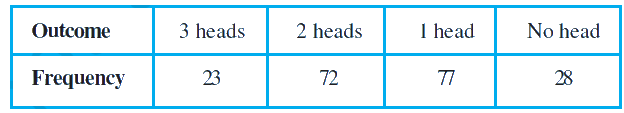If the three coins are simultaneously tossed again, compute the probability of $$2$$ heads coming up.

### Solution

What is known?

Total number of tosses and frequencies of different outcomes.

What is unknown?

Probability of $$2$$ heads coming up when three coins are tossed simultaneously.

Reasoning:

The empirical probability $$P(E)$$ of an event $$E$$ happening, is given by:

\begin{align}{P}({E})=\frac{ \begin{bmatrix} \text { Number of trials in which }\\ \text{the event happened } \end{bmatrix} }{ \begin{bmatrix} \text { The total number} \\ \text{of trials } \end{bmatrix} }\end{align}

Use probability to derive the solution where

Probability of a particular outcome

\begin{align} & { = \frac{\begin{bmatrix}{\text{No of time of a particular }} \\ {\text{outcome occurs }}\end{bmatrix} }{{{\text{Total number of tosses}}}}} \end{align}

Steps:

Given total number of tosses $$= 200$$

No of $$2$$ heads outcomes $$= 72$$

Probability [of head outcomes]

\begin{align} & { = \frac{ {\text{Number of times }} {\text{heads occur}} }{{{\text{Total number of tosses}}}}} \\ &=\frac{72}{200} \\ &=\frac{9}{25} \end{align}

## Chapter 15 Ex.15.1 Question 5

An organization selected $$2400$$ families at random and surveyed them to determine a relationship between income level and the number of vehicles in a family. The information gathered is listed in the table below.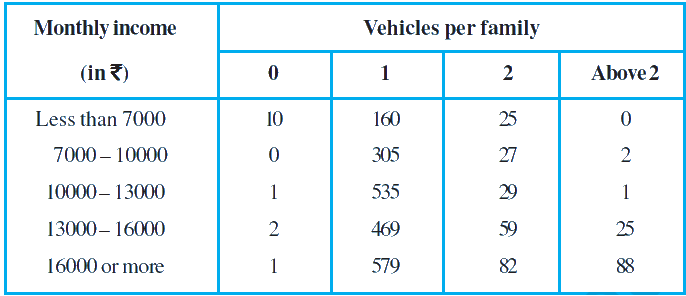Suppose a family is chosen. Find the probability that the family chosen is

(i) earning $$\rm Rs 10000 – 13000$$ per month and owning exactly $$2$$ vehicles.

(ii) earning $$\rm Rs 16000$$ or more per month and owning exactly $$1$$ vehicle.

(iii) earning less than $$\rm Rs 7000$$ per month and does not own any vehicle.

(iv) earning $$\rm Rs 13000 – 16000$$ per month and owning more than $$2$$ vehicles.

(v) owning not more than $$1$$ vehicle.

### Solution

What is known?

Family monthly income and vehicles per family.

What is unknown?

Probability of family owning $$0$$ or $$1$$ or $$2$$ or above $$2$$ vehicles based on the incomes.

Reasoning:

The empirical probability $$P(E)$$ of an event $$E$$ happening, is given by:

\begin{align} & { = \frac{ {\text{Number of times }} {\text{heads occur}} }{{{\text{Total number of tosses}}}}} \\ &=\frac{72}{200} \\ &=\frac{9}{25} \end{align}

Use probability to derive the solution where

Probability (of family owning vehicle based on the earnings)

\begin{align} = \frac{ \begin{bmatrix} \text{No of vehicles owned} \\ \text{ based on earnings} \end{bmatrix} }{ \text{Total number} \\ \text{ of families} } \end{align}

Steps:

Total no of families $$= 2400$$

(i)

Probability $$P1$$ (a family earning $$\rm Rs 13000$$ to $$\rm Rs 10000$$ per month and owning exactly $$2$$ vehicles)

\begin{align} &=\!\frac{ \begin{bmatrix} \text{No of a families earning Rs 13000}\\\text{to Rs 10000 per month and} \\ \text{owning exactly 2 vehicles} \end{bmatrix} }{{{\text{Total number of families}}}}\\& = \,\frac{{{\rm{29}}}}{{{\rm{2400}}}}\end{align}

(ii)

Probability $$P2$$ (a family earning $$\rm Rs 16000$$ per month and owning exactly $$1$$ vehicle)

\begin{align} &=\frac{ \begin{bmatrix} \text{No of a families earning}\\\text{ Rs 16000 per month and }\\\text{owning exactly 1 vehicle} \end{bmatrix} }{{{\text{Total number of families}}}}\\ \\ &= \frac{{{\rm{579}}}}{{{\rm{2400}}}}\, = \frac{{193}}{{800}}\end{align}

(iii)

Probability $$P3$$ (a family earning less than $$\rm Rs 7000$$ per month and does not own any vehicle)

\begin{align}& =\! \frac{\begin{bmatrix} \text{No of a families earning} \\\text{less than Rs 7000 per month} \\ \text{and does not own any vehicle} \end{bmatrix} }{{{\text{Total number of families}}}}\\ \\ &= \,\frac{{10}}{{{\rm{2400}}}}\\&= \frac{1}{{240}}\end{align}

(iv)

Probability $$P4$$ (a family earning Rs $$13000$$ to Rs $$16000$$ per month and owning more than $$2$$ vehicles)

\begin{align} &= \frac{ \begin{bmatrix} \text{No of a families earning } \\ \text{Rs 16000 to Rs 13000 per } \\ \text{month and owning more }\\\text{ than 2 vehicles}\end{bmatrix} }{{{\text{Total number of families}}}}\\ & = \,\frac{{{\rm{25}}}}{{{\rm{2400}}}} = \frac{1}{{96}}\end{align}

(v)

Probability $$P5$$ (a family owning $$0$$ or $$1$$ vehicle)

Total number of families

\begin{align} & = \,\frac{\begin{bmatrix} 10 + 0 + 1 + 2 + 1 + 160 \\ + 305 + 535 + 469 + 579 \end{bmatrix} }{{{\rm{2400}}}} \\ \\ &= \frac{{2062}}{{2400}} = \frac{{1031}}{{1200}}\end{align}

## Chapter 15 Ex.15.1 Question 6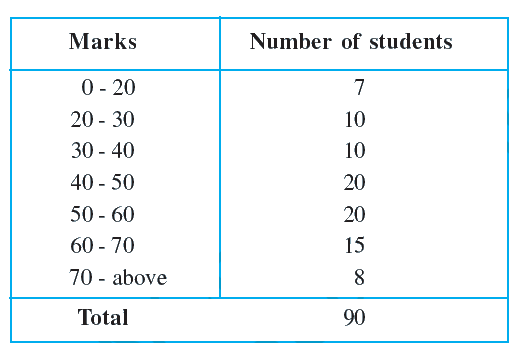(i) Find the probability that a student obtained less than $$20\%$$ in the mathematics test.

(ii) Find the probability that a student obtained $$60$$ marks or above.

### Solution

What is known?

Marks in different range and respective students.

What is unknown?

Probability of students obtained less than $$20\%$$ in math. Probability of students obtained $$60$$ marks or above.

Reasoning:

The empirical probability $$P(E)$$ of an event $$E$$ happening, is given by:

\begin{align}{P}({E})=\frac{ \begin{pmatrix} \text { Number of trials in which }\\ \text{the event happened } \end{pmatrix} }{ \text { The total number of trials } }\end{align}

Use probability to derive the solution where

Probability (students in range of marks)

\begin{align}=\frac{ \begin{pmatrix} \text { Number of students} \\ \text{ in range of marks } \end{pmatrix}}{\text { Total number of students }} \end{align}

Steps:

Total no of students $$= 90$$

(i)

Probability (a student obtained less than $$20\%$$)

\begin{align} &= \frac{ \begin{pmatrix} \text{No of students who} \\ \text{ obtained less than 20% } \end{pmatrix}}{{{\text{Total number of students}}}} \\ &= \frac{7}{90} \end{align}

(ii)

Probability (a student obtained $$60$$ marks or above)

\begin{align} &= \frac{ \begin{pmatrix}\text{No of students obtained } \\ \text{60 marks or above} \end{pmatrix}}{{{\text{Total number of students}}}} \\ &= \frac{ (15+8)}{90} =\frac{ 23}{90} \end{align}

## Chapter 15 Ex.15.1 Question 7

To know the opinion of the students about the subject statistics, a survey of $$200$$ students was conducted. The data is recorded in the following table.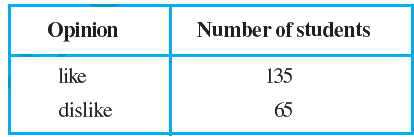Find the probability that a student chosen at random

(i) likes statistics,

(ii) does not like it.

### Solution

What is known?

Number of students who like statistics and who do not like statistics.

What is the unknown?

Probability of number of students who like statistics and who do not like statistics.

Reasoning:

The empirical probability $$P(E)$$ of an event E happening, is given by:

\begin{align}{P}({E})=\frac{ \begin{pmatrix} \text { Number of trials in which }\\ \text{the event happened } \end{pmatrix} }{ \text { The total number of trials } }\end{align}

Use probability to derive the solution where

Probability (students like/dislike statistics)

\begin{align}=\frac{ \begin{pmatrix} \text { Number of students who } \\ \text{like or dislike statistics } \end{pmatrix} }{\text { Total number of students }}\end{align}

Steps:

Total no of students $$= 200$$

(i) Likes statistics

Probability students like statistics

\begin{align}&\text{ = }\frac{\text{No of students like statistics }}{\text{ Total no of students}} \\ & \text{= }\frac{135}{200}\text{=}\frac{27}{40} \end{align}

(ii) Does not likes statistics

Probability students dislike statistics

\begin{align}&\text{ = }\frac{\text{No of students dislike statistics }}{\text{ Total no of students}} \\ & \text{= }\frac{65}{200}\text{=}\frac{13}{40} \end{align}

## Chapter 15 Ex.15.1 Question 8

The distance (in km) of $$40$$ engineers from their residence to their place of work were found as follows: What is the empirical probability that an engineer lives:

 $$5$$ $$3$$ $$10$$ $$20$$ $$25$$ $$11$$ $$13$$ $$7$$ $$12$$ $$31$$ $$19$$ $$10$$ $$12$$ $$17$$ $$18$$ $$11$$ $$32$$ $$17$$ $$16$$ $$2$$ $$7$$ $$9$$ $$7$$ $$8$$ $$3$$ $$5$$ $$12$$ $$15$$ $$18$$ $$3$$ $$12$$ $$14$$ $$2$$ $$9$$ $$6$$ $$15$$ $$15$$ $$7$$ $$6$$ $$12$$

(i) less than $$7 \,\rm km$$ from her place of work?

(ii) more than or equal to $$7\,\rm km$$ from her place of work?

(iii) within $$½\,\rm km$$ from her place of work?

### Solution

What is known?

No of engineers and distance of their work place to the residence.

What is unknown?

Probability of distance of work place of engineers from their residence.

Reasoning:

The empirical probability $$P(E)$$ of an event $$E$$ happening, is given by:

\begin{align}{P}({E})=\frac{ \begin{pmatrix} \text { Number of trials in which }\\ \text{the event happened } \end{pmatrix} }{ \text { The total number of trials } }\end{align}

Use probability to derive the solution where

Probability (no of engineers travelling certain distance from residence to their place of work)

\begin{align}&\\ &=\frac{\text { Number of engineers }}{\text { Total number of engineers }}\end{align}

Steps:

Total no of engineers $$= 40$$

By arranging the numbers in increasing order

$$2, 2, 3, 3, 3, 5, 5, 6, 6, 7, 7, 7,\\ 7, 8, 9, 9, 10, 10, 11, 11, 12, \\ 12, 12, 12, 12, 13, 14, 15, 15, \\ 15, 16,17, 17, 18, 18, 19, 20, \\ 25, 31, 32.$$

No. of engineers who live less than $$7\,\rm km$$ from their place of work $$= 9$$

No. of engineers who live more than or equal to $$7\,\rm km$$ from their work place $$= 31$$

No. of engineers who live within $$½ \,\rm km$$ from their place of work$$=0$$

(i)

Probability $$P1$$(An engineer lives less than $$7\,\rm km$$ from their work place)

\begin{align}\\&=\frac{ \begin{pmatrix} \text { Number of engineers who} \\ \text{ live less than 7 km } \\ \text{from their work place } \end{pmatrix} }{\text { Total number of engineers }}\\ &=\frac{9}{40}\end{align}

(ii)

Probability $$P2$$(An engineer lives more than or equal to $$7\,\rm km$$ from their work place)

\begin{align}\\&=\frac{ \begin{pmatrix} \text { Number of engineers who} \\ \text{ live more than or equal to} \\ 7 \text{ km from their work place } \end{pmatrix} }{\text { Total number of engineers }}\\ &=\frac{31}{40}\end{align}

(iii)

Probability $$P3$$(An engineer lives within $$1/2\,\rm km$$ from their work place)

\begin{align}\\&=\frac{ \begin{pmatrix} \text { Number of engineers who }\\ \text{ live within 1/2 km } \\ \text{from their work place } \end{pmatrix}}{\text { Total number of engineers }}\\ &=\frac{0}{40}=0\end{align}

## Chapter 15 Ex.15.1 Question 9

Eleven bags of wheat flour, each marked $$5\,\rm kg$$, actually contained the following weights of flour (in kg)

$$4.97, 5.05, 5.08, 5.03,\\ 5.00, 5.06, 5.08,\\ 4.98, 5.04, 5.07, 5.00$$

Find the probability that any of these bags chosen at random contains more than $$5 \,\rm kg$$ of flour.

### Solution

What is known?

No of bags of specific weights.

What is unknown?

Probability of any of the bags containing more than $$5\,\rm kg$$ of flour.

Reasoning:

The empirical probability $$P(E)$$ of an event $$E$$ happening, is given by:

\begin{align}{P}({E})=\frac{ \begin{pmatrix} \text { Number of trials in which }\\ \text{the event happened } \end{pmatrix} }{ \text { The total number of trials } }\end{align}

Use probability to derive the solution where

Probability $$P$$ Weight of the bag

\begin{align}=\frac{ \begin{pmatrix} \text { Number of bags} \\ \text{ of specific weight } \end{pmatrix} }{\text { Total number of bags }}\end{align}

Steps:

Total no of bags $$= 11$$

No of bags more than $$5\,\rm kg$$ of flour $$= 7$$

Probability (Bag weighing more than $$5\,\rm kg$$ of flour )

\begin{align}\\&=\frac{ \begin{pmatrix} \text { Number of bags weighing} \\ \text{ more than 5 kg of flour } \end{pmatrix}} {\text { Total number of bags }} \\ &=\frac{7}{11}\end{align}

## Chapter 15 Ex.15.1 Question 10

A study was conducted to find out the concentration of sulphur dioxide in the air parts per million ($$\rm ppm$$) of a certain city. The data obtained for $$30\,\rm days$$ is as follows.

 $$0.03$$ $$0.08$$ $$0.08$$ $$0.09$$ $$0.04$$ $$0.17$$ $$0.16$$ $$0.05$$ $$0.02$$ $$0.06$$ $$0.18$$ $$0.2$$ $$0.11$$ $$0.08$$ $$0.12$$ $$0.13$$ $$0.22$$ $$0.07$$ $$0.08$$ $$0.01$$ $$0.1$$ $$0.06$$ $$0.09$$ $$0.18$$ $$0.11$$ $$0.07$$ $$0.05$$ $$0.07$$ $$0.01$$ $$0.04$$

find the probability of the concentration of sulphur dioxide in the interval $$0.12 - 0.16$$ on any of these days.

### Solution

What is known

Concentration of sulphur dioxide for $$30$$ days.

What is unknown?

Probability of the concentration of sulphur dioxide in the interval $$0.12 - 0.16.$$

Reasoning:

The empirical probability $$P(E)$$ of an event $$E$$ happening, is given by:

\begin{align}{P}({E})=\frac{ \begin{pmatrix} \text { Number of trials in which }\\ \text{the event happened } \end{pmatrix} }{ \text { The total number of trials } }\end{align}

Use probability to derive the solution where

Probability (Concentration of Sulphur dioxide )

\begin{align}=\frac{ \begin{pmatrix} \text { Number of days of} \\ \text{ specific concentration } \end{pmatrix} }{\text { Total number of days }} \end{align}

Steps:

Total no of days $$= 30$$

No of days on which concentration was in the interval $$0.12-0.16 = 2$$

Probability (Concentration of Sulphur dioxide in the interval $$0.12 - 0.16$$)

\begin{align}\\&=\frac{ \begin{pmatrix} \text { Number of days when } \\ \text{ concentration was in} \\ \text{ the interval } 0.12-0.16 \end{pmatrix} }{\text { Total number of days }} \\ &=\frac{2}{30}=\frac{1}{15}\end{align}

## Chapter 15 Ex.15.1 Question 11

The blood groups of $$30$$ students of Class VIII are recorded as follows:

$$A,\, B, \,O,\, O,\, AB,\, O,\, A,\, O,\,\\ B,\, A,\, O,\, B,\, A,\, O,\, O,\, A,\,\\ AB,\, O,\, A,\, A,\, O,\, O,\, AB,\,\\ B,\, A,\, O,\, B,\, A,\, B,\, O.$$

Use this table to determine the probability that a student of this class, selected at random, has blood group $$AB.$$

### Solution

What is known?

No of students and their blood group.

What is unknown?

Probability of students having blood group $$AB.$$

Reasoning:

The empirical probability $$P(E)$$ of an event $$E$$ happening, is given by:

\begin{align}{P}({E})=\frac{ \begin{pmatrix} \text { Number of trials in which }\\ \text{the event happened } \end{pmatrix} }{ \text { The total number of trials } }\end{align}

Use probability to derive the solution where

Probability (Of a selected student having a specific blood group)

\begin{align}\\&=\frac{ \begin{pmatrix}\text { Number of students having} \\ \text{ specific blood group} \end{pmatrix}}{\text { Total number of students }} \end{align}

Steps:

Total no of students $$= 30$$

No of students having blood group $$AB = 3$$

Probability $$P$$ (Of a student having blood group $$AB$$)

\begin{align}\\&=\frac{ \begin{pmatrix} \text { Number of students having} \\ \text{ blood group } AB \end{pmatrix} }{\text { Total number of students }} \\ &=\frac{3}{30} \\& =\frac{1}{10} \end{align}

Instant doubt clearing with Cuemath Advanced Math Program